# X axis lables missing when using Javascript (HTML5) vs Flash for RealTimeArea

## Recommended Posts

hello,

I have a series of webpages that use RealTimeArea.swf to graph the number of calls coming in.

We have been trying to move over to using the Javascript (HTML5) rendering engine, so that more browsers can show the graphs for our clients, but we still have to support IE8 and 9.

I have enclosed the code that is used to create and give the chart some data. it is the same for all instances of our web page.

we use:

<script type=""text/javascript"">FusionCharts.setCurrentRenderer('javascript');</script>

when we want the charts to be forced over to javascript rendering.

The issue we are having is that the category labels on the x-axis are not always showing. I can see in the data that I have them, but the HTML5 renderer does not show them.  The flash version does.

any ideas why?

thanks

Geordi

fusion chart code.txt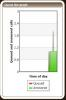##### Share on other sitesHi,

Could you please let us know the version of FusionWidgets XT you are using. (You can find the version details by opening the FusionCharts.js file in any text editor and look for first few commented lines)?

If it is not the latest FusionWidgets XT v3.3.1sr3, please try once by replacing the latest trial JavaScript files into your application.

##### Share on other sites

we are using fusioncharts/3.3.1-sr3.21100, which was the latest that I could download this week.

##### Share on other sites

we are using fusioncharts/3.3.1-sr3.21100, which was the latest that I could download this week.

Hi,

We are unable to replicate the issue with the Realtime charts from the FusionWidgets XT (v3.3.1) Service Release 3. You may check with the samples on our website for reference.

In case this does not help, please provide us with the XML/JSON data along with the URL from where the data is fed, so that we can test it.

Hope this helps.##### Share on other sites

the code that we used to create the chart is in the file fusion chart code.txt.  the data is feed in by javascript updates to the webpage.  I will do some checking and testing on Monday and get back to you, and try to see if I can recreate it in a simpler page layout.

##### Share on other sites

I have managed to recreate the issue, and have enclosed the 3 html files and images of the issue.

1. sample swf original code.html

This works as expected and I see all the labels on the x-axis

<div id="chartContainer">FusionCharts XT will load here!</div>
<script type="text/javascript"><!--

var myChart = new FusionCharts( "Fusion/FusionWidgets_XT_Professional/Charts/RealTimeArea.swf",
"myChartId", "400", "300", "0", "1" );

2. sample javascrip via modified swf original code.html

This only shows the 1st and last x labels.  it is missing 09:45, 10:00 and 10;15

<div id="chartContainer">FusionCharts XT will load here!</div>
<script type="text/javascript"><!--

var myChart = new FusionCharts( "RealTimeArea",
"myChartId", "400", "300");

3. sample pure javascrip.html

this is the same as above, as it is missing most of the x axis labels.

<div id="chartContainer">FusionCharts XT will load here!</div>
<script type="text/javascript"><!--

FusionCharts.setCurrentRenderer('javascript');

var myChart = new FusionCharts( "Fusion/FusionWidgets_XT_Professional/Charts/RealTimeArea.swf",
"myChartId", "400", "300", "0", "1" );

I recreated this all by using one of your examples and modifying it to how we are using it.

first we put the inital html and javascript from our server, with the initial data, in a javascript string.

we then update via javascript from our server.

var stChartConfig = '';
stChartConfig += "";
stChartConfig += "<Chart ";
stChartConfig += "    showBorder='0'";
stChartConfig += "    baseFontSize  ='12'";
stChartConfig += "    baseFontColor ='000000'";
stChartConfig += "    lowerLimit='0' ";
stChartConfig += "    yAxisName = 'Queued and answered calls' ";
....
stChartConfig += ">";
stChartConfig += "   <categories> ";
stChartConfig += "   <category label='09:30' />";
stChartConfig += "   <category label=' ' />";
....
stChartConfig += "   </categories> ";
stChartConfig += "   <dataset ";
stChartConfig += "       seriesName='Queued' ";
stChartConfig += "       color='#FF0000' ";
stChartConfig += "   >";
stChartConfig += "   <set value='0' />";
....
stChartConfig += "   </dataset>";
stChartConfig += "   <dataset ";
stChartConfig += "       color='#00FF00' ";
stChartConfig += "       showPlotBorder = '0' ";
stChartConfig += "   >";
stChartConfig += "   <set value='0' />";
....
stChartConfig += "   <set value='1' />";
stChartConfig += "   </dataset>";
stChartConfig += "";
stChartConfig += "   <styles>";
stChartConfig += "       <definition>";
stChartConfig += "           <style type='font' name='captionFont' size='16' color='000000' />";
stChartConfig += "       </definition>";
stChartConfig += "       <application>";
stChartConfig += "           <apply toObject='Caption' styles='captionFont' />";
stChartConfig += "       </application>";
stChartConfig += "   </styles>";
stChartConfig += "</Chart>";

myChart.setXMLData(stChartConfig);
myChart.render("chartContainer");

Can you please explain why we are missing some of the x-axis labels?

thanks
Geordi

sample javascrip via modified swf original code.html

sample pure javascrip.html

sample swf original code.html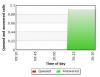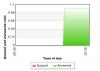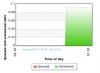##### Share on other sitesHi,

We are looking into your issue and the files provided.

We will get back to you with the updates on this, at the earliest.

##### Share on other sites

FYI,

This is similar issue to my recent post on xAxis labels not showing in Javascript PhP for LogMSColumn2d.swf

##### Share on other sitesHi,

It seems in your generated XML code, you are providing two <set> elements in a <dataset></dataset> whereas, there is only one <category/> element present.

Please note that in multi-series data format, the number of each data set elements should be equal to the number of categories provided in that chart.

For more details on "Real-time Area Chart Specification Sheet ", please visit the link: http://docs.fusioncharts.com/widgets/Contents/DataStreaming/XMLRealTimeArea.html

Hope this helps!

##### Share on other sites

hello,

<html>
<title>My First chart using FusionCharts XT - Using pure JavaScript</title>
<script type="text/javascript" src="Fusion/FusionWidgets_XT_Professional/Charts/FusionCharts.js"></script>
<body>
<div id="chartContainer">FusionCharts XT will load here!</div>
<script type="text/javascript"><!--

FusionCharts.setCurrentRenderer('javascript');

var myChart = new FusionCharts( "Fusion/FusionWidgets_XT_Professional/Charts/RealTimeArea.swf",
"myChartId", "400", "300", "0", "1" );
var stChartConfig = '';
stChartConfig += "";
stChartConfig += "<Chart ";
stChartConfig += "    showBorder='0'";
stChartConfig += "    baseFontSize  ='12'";
stChartConfig += "    baseFontColor ='000000'";
stChartConfig += "    lowerLimit='0' ";
stChartConfig += "    yAxisName = 'Queued and answered calls' ";
stChartConfig += "    xAxisName = 'Time of day'";
stChartConfig += "    showRealTimeValue = '0' ";
stChartConfig += "    drawAnchors = '0' ";
stChartConfig += "     ";
stChartConfig += "    showValues='0' ";
stChartConfig += "    labelDisplay='Rotate' ";
stChartConfig += "    numDisplaySets='63'";
stChartConfig += "    canvasBottomMargin='0' ";
stChartConfig += "    bgColor='FFFFFF' ";
stChartConfig += "     ";
stChartConfig += ">";
stChartConfig += "   <categories> ";
stChartConfig += "   <category label='09:30' />";
stChartConfig += "   <category label=' ' />";
stChartConfig += "   <category label=' ' />";
stChartConfig += "   <category label=' ' />";
stChartConfig += "   <category label=' ' />";
stChartConfig += "   <category label=' ' />";
stChartConfig += "   <category label=' ' />";
stChartConfig += "   <category label=' ' />";
stChartConfig += "   <category label=' ' />";
stChartConfig += "   <category label=' ' />";
stChartConfig += "   <category label=' ' />";
stChartConfig += "   <category label=' ' />";
stChartConfig += "   <category label=' ' />";
stChartConfig += "   <category label=' ' />";
stChartConfig += "   <category label=' ' />";
stChartConfig += "   <category label='09:45' />";
stChartConfig += "   <category label=' ' />";
stChartConfig += "   <category label=' ' />";
stChartConfig += "   <category label=' ' />";
stChartConfig += "   <category label=' ' />";
stChartConfig += "   <category label=' ' />";
stChartConfig += "   <category label=' ' />";
stChartConfig += "   <category label=' ' />";
stChartConfig += "   <category label=' ' />";
stChartConfig += "   <category label=' ' />";
stChartConfig += "   <category label=' ' />";
stChartConfig += "   <category label=' ' />";
stChartConfig += "   <category label=' ' />";
stChartConfig += "   <category label=' ' />";
stChartConfig += "   <category label=' ' />";
stChartConfig += "   <category label='10:00' />";
stChartConfig += "   <category label=' ' />";
stChartConfig += "   <category label=' ' />";
stChartConfig += "   <category label=' ' />";
stChartConfig += "   <category label=' ' />";
stChartConfig += "   <category label=' ' />";
stChartConfig += "   <category label=' ' />";
stChartConfig += "   <category label=' ' />";
stChartConfig += "   <category label=' ' />";
stChartConfig += "   <category label=' ' />";
stChartConfig += "   <category label=' ' />";
stChartConfig += "   <category label=' ' />";
stChartConfig += "   <category label=' ' />";
stChartConfig += "   <category label=' ' />";
stChartConfig += "   <category label=' ' />";
stChartConfig += "   <category label='10:15' />";
stChartConfig += "   <category label=' ' />";
stChartConfig += "   <category label=' ' />";
stChartConfig += "   <category label=' ' />";
stChartConfig += "   <category label=' ' />";
stChartConfig += "   <category label=' ' />";
stChartConfig += "   <category label=' ' />";
stChartConfig += "   <category label=' ' />";
stChartConfig += "   <category label=' ' />";
stChartConfig += "   <category label=' ' />";
stChartConfig += "   <category label=' ' />";
stChartConfig += "   <category label=' ' />";
stChartConfig += "   <category label=' ' />";
stChartConfig += "   <category label=' ' />";
stChartConfig += "   <category label=' ' />";
stChartConfig += "   <category label='10:30' />";
stChartConfig += "   <category label=' ' />";
stChartConfig += "   <category label=' ' />";
stChartConfig += "   </categories> ";
stChartConfig += "   <dataset ";
stChartConfig += "       seriesName='Queued' ";
stChartConfig += "       color='#FF0000' ";
stChartConfig += "   >";
stChartConfig += "   <set value='0' />";
stChartConfig += "   <set value='0' />";
stChartConfig += "   <set value='0' />";
stChartConfig += "   <set value='0' />";
stChartConfig += "   <set value='0' />";
stChartConfig += "   <set value='0' />";
stChartConfig += "   <set value='0' />";
stChartConfig += "   <set value='0' />";
stChartConfig += "   <set value='0' />";
stChartConfig += "   <set value='0' />";
stChartConfig += "   <set value='0' />";
stChartConfig += "   <set value='0' />";
stChartConfig += "   <set value='0' />";
stChartConfig += "   <set value='0' />";
stChartConfig += "   <set value='0' />";
stChartConfig += "   <set value='0' />";
stChartConfig += "   <set value='0' />";
stChartConfig += "   <set value='0' />";
stChartConfig += "   <set value='0' />";
stChartConfig += "   <set value='0' />";
stChartConfig += "   <set value='0' />";
stChartConfig += "   <set value='0' />";
stChartConfig += "   <set value='0' />";
stChartConfig += "   <set value='0' />";
stChartConfig += "   <set value='0' />";
stChartConfig += "   <set value='0' />";
stChartConfig += "   <set value='0' />";
stChartConfig += "   <set value='0' />";
stChartConfig += "   <set value='0' />";
stChartConfig += "   <set value='0' />";
stChartConfig += "   <set value='0' />";
stChartConfig += "   <set value='0' />";
stChartConfig += "   <set value='0' />";
stChartConfig += "   <set value='0' />";
stChartConfig += "   <set value='0' />";
stChartConfig += "   <set value='0' />";
stChartConfig += "   <set value='0' />";
stChartConfig += "   <set value='0' />";
stChartConfig += "   <set value='0' />";
stChartConfig += "   <set value='0' />";
stChartConfig += "   <set value='0' />";
stChartConfig += "   <set value='1' />";
stChartConfig += "   <set value='1' />";
stChartConfig += "   <set value='1' />";
stChartConfig += "   <set value='1' />";
stChartConfig += "   <set value='1' />";
stChartConfig += "   <set value='1' />";
stChartConfig += "   <set value='1' />";
stChartConfig += "   <set value='1' />";
stChartConfig += "   <set value='1' />";
stChartConfig += "   <set value='1' />";
stChartConfig += "   <set value='1' />";
stChartConfig += "   <set value='1' />";
stChartConfig += "   <set value='1' />";
stChartConfig += "   <set value='1' />";
stChartConfig += "   <set value='1' />";
stChartConfig += "   <set value='1' />";
stChartConfig += "   <set value='1' />";
stChartConfig += "   <set value='1' />";
stChartConfig += "   <set value='1' />";
stChartConfig += "   <set value='1' />";
stChartConfig += "   <set value='1' />";
stChartConfig += "   <set value='1' />";
stChartConfig += "   </dataset>";
stChartConfig += "   <dataset ";
stChartConfig += "       color='#00FF00' ";
stChartConfig += "       showPlotBorder = '0' ";
stChartConfig += "   >";
stChartConfig += "   <set value='0' />";
stChartConfig += "   <set value='0' />";
stChartConfig += "   <set value='0' />";
stChartConfig += "   <set value='0' />";
stChartConfig += "   <set value='0' />";
stChartConfig += "   <set value='0' />";
stChartConfig += "   <set value='0' />";
stChartConfig += "   <set value='0' />";
stChartConfig += "   <set value='0' />";
stChartConfig += "   <set value='0' />";
stChartConfig += "   <set value='0' />";
stChartConfig += "   <set value='0' />";
stChartConfig += "   <set value='0' />";
stChartConfig += "   <set value='0' />";
stChartConfig += "   <set value='0' />";
stChartConfig += "   <set value='0' />";
stChartConfig += "   <set value='0' />";
stChartConfig += "   <set value='0' />";
stChartConfig += "   <set value='0' />";
stChartConfig += "   <set value='0' />";
stChartConfig += "   <set value='0' />";
stChartConfig += "   <set value='0' />";
stChartConfig += "   <set value='0' />";
stChartConfig += "   <set value='0' />";
stChartConfig += "   <set value='0' />";
stChartConfig += "   <set value='0' />";
stChartConfig += "   <set value='0' />";
stChartConfig += "   <set value='0' />";
stChartConfig += "   <set value='0' />";
stChartConfig += "   <set value='0' />";
stChartConfig += "   <set value='0' />";
stChartConfig += "   <set value='0' />";
stChartConfig += "   <set value='0' />";
stChartConfig += "   <set value='0' />";
stChartConfig += "   <set value='0' />";
stChartConfig += "   <set value='0' />";
stChartConfig += "   <set value='0' />";
stChartConfig += "   <set value='0' />";
stChartConfig += "   <set value='0' />";
stChartConfig += "   <set value='0' />";
stChartConfig += "   <set value='0' />";
stChartConfig += "   <set value='1' />";
stChartConfig += "   <set value='1' />";
stChartConfig += "   <set value='1' />";
stChartConfig += "   <set value='1' />";
stChartConfig += "   <set value='1' />";
stChartConfig += "   <set value='1' />";
stChartConfig += "   <set value='1' />";
stChartConfig += "   <set value='1' />";
stChartConfig += "   <set value='1' />";
stChartConfig += "   <set value='1' />";
stChartConfig += "   <set value='1' />";
stChartConfig += "   <set value='1' />";
stChartConfig += "   <set value='1' />";
stChartConfig += "   <set value='1' />";
stChartConfig += "   <set value='1' />";
stChartConfig += "   <set value='1' />";
stChartConfig += "   <set value='1' />";
stChartConfig += "   <set value='1' />";
stChartConfig += "   <set value='1' />";
stChartConfig += "   <set value='1' />";
stChartConfig += "   <set value='1' />";
stChartConfig += "   <set value='1' />";
stChartConfig += "   </dataset>";
stChartConfig += "";
stChartConfig += "   <styles>";
stChartConfig += "       <definition>";
stChartConfig += "           <style type='font' name='captionFont' size='16' color='000000' />";
stChartConfig += "       </definition>";
stChartConfig += "       <application>";
stChartConfig += "           <apply toObject='Caption' styles='captionFont' />";
stChartConfig += "       </application>";
stChartConfig += "   </styles>";
stChartConfig += "</Chart>";

myChart.setXMLData(stChartConfig);
myChart.render("chartContainer");

// -->
</script>
</body>
</html>

I saw this in the page that you referred me to:

In multi-series charts, it's necessary to provide an equal number of <category> elements and data items (<set> elements within each <dataset>).

there was one <categories> with 63 <category> elements, and 2 <datasets> with 63 <set> elements each.  so based on what is says, I did it correctly.  did you look at 3 html files that i included?  they all had 63 elements in each <categories> and <dataset>. based on your comment, it seems you where looking at my cut down version for posting:

stChartConfig += "   <categories> ";
stChartConfig += "   <category label='09:30' />";
stChartConfig += "   <category label=' ' />";
....
stChartConfig += "   </categories> ";
stChartConfig += "   <dataset ";
stChartConfig += "       seriesName='Queued' ";
stChartConfig += "       color='#FF0000' ";
stChartConfig += "   >";
stChartConfig += "   <set value='0' />";
....
stChartConfig += "   </dataset>";
stChartConfig += "   <dataset ";
stChartConfig += "       color='#00FF00' ";
stChartConfig += "       showPlotBorder = '0' ";
stChartConfig += "   >";
stChartConfig += "   <set value='0' />";
....
stChartConfig += "   <set value='1' />";
stChartConfig += "   </dataset>";

were I had removed lines and just put the "...." for continuation.

or must I have a <categories> with 63 elements for every <dataset> with 63 elements?  I tried that, and I am still missing the x-axis labels.

thanks##### Share on other sites

here is a copy of my test file:

Copy of sample pure javascrip.html

##### Share on other sites

Hi,

You can upgrade to the latest version of FusionCharts Suite XT, it has greater support for JavaScript charts and all your labels will be displayed in the chart properly. Please find the screenshot attached.

If you would like to use older versions then try the following:

```<chart ...labelDisplay='NONE' rotateLabels='1'>
```

Hope this helps,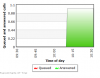##### Share on other sites

thanks for the fix. it is working on 3.3.1 sr3

I could not find a download version of 3.4 to try with.

Regards

G Sinclair